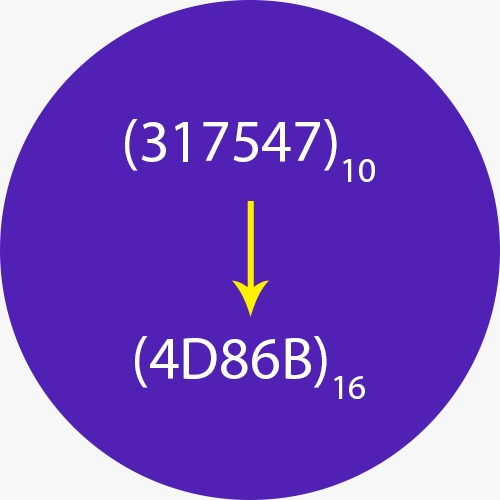﻿ Decimal to Hex Converter | Decimal to Hexadecimal Calculator

# Decimal to Hex Calculator

Use our Decimal to Hexadecimal converter a free online calculator / conversion tool to convert decimal value into hex number system.

#### Decimal Number System

Decimal number system has only ten (10) digits from 0 to 9. Every number (value) represents with 0,1,2,3,4,5,6,7,8 and 9 in this number system. The base of decimal number system is 10, because it has only 10 digits

A Hexadecimal number system has sixteen (16) alphanumeric values from 0 to 9 and A to F. Every number (value) represents with 0,1,2,3,4,5,6,7,8,9,A,B,C,D,E and F in this number system. The base of hexadecimal number system is 16, because it has 16 alphanumeric values. Here A is 10, B is 11, C is 12, D is 14, E is 15 and F is 16.0 0000 0 0
1 0001 1 1
2 0010 2 2
3 0011 3 3
4 0100 4 4
5 0101 5 5
6 0110 6 6
7 0111 7 7
8 1000 10 8
9 1001 11 9
10 1010 12 A
11 1011 13 B
12 1100 14 C
13 1101 15 D
14 1110 16 E
15 1111 17 F